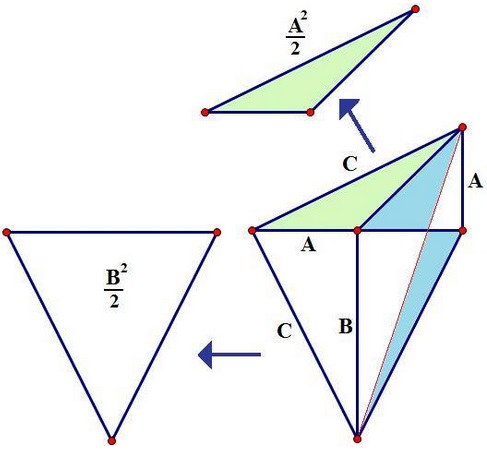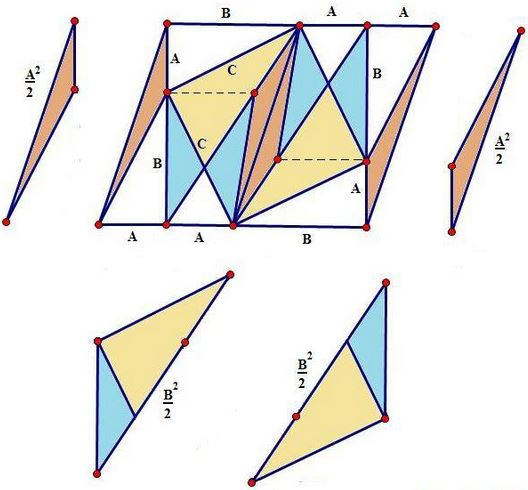# Pythagoras, with a Nod to the Carpets Theorem

The proofs below are by Tony Foster, III.

### Proof 1

This is the simplest of the three and has been submitted last. It is based on the following diagramIn the diagram, the side length of the triangles are unconventionally denoted by capital letters. The two triangles, one of area $\displaystyle\frac{A^2}{2},$ the other of area $\displaystyle\frac{B^2}{2},$ have been duplicated separately from the main figure. The clue to the proof is twofold: first locate the right isosceles triangle with legs $C$ and then observe that the two blue triangles have the same area. This is a very general property of trapezoids that has been established in the proof of the Carpets Theorem. The diagram leads to the required identity: $\displaystyle\frac{A^2}{2}+\frac{B^2}{2}=\frac{C^2}{2}.$

### Proof 2

The second proof is a little more complicated. Note that $\Delta KMO$ is right isosceles with the legs of length $C.$By the property of trapezoids already used in Proof 1, triangles $NOQ$ and $MPQ$ have equal areas. More than that, triangles $JLN$ and $KNM$ have equal areas as are triangles $OLN$ and $PNM$ so that this is also true of the pair $OLN$ and $PNM,$ making the remaining triangles $JLO$ and $KPM$ of equal area. So we have

\displaystyle\begin{align} \frac{C^2}{2}&=Area(\Delta KOM)\\ &=Area(\Delta KOQ + \Delta MPQ +\Delta KPM)\\ &=Area(\Delta KOQ) + Area(\Delta MPQ) +Area(\Delta KPM)\\ &=Area(\Delta KOQ) + Area(\Delta NOQ) +Area(\Delta JLO)\\ &=Area(\Delta KNO) + frac{A^2}{2}\\ &=\frac{B^2}{2} + \frac{A^2}{2}. \end{align}

### Proof 3

This proof is the symmetric variant of the previous one. It is based on the following diagram